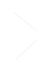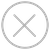TensorSpace.jsTensorSpace Converter``````let model = new TSP.models.Sequential( container );
initStatus: "open"
}) );
outputs: ["0", "1", "2", "3", "4", "5", "6", "7", "8", "9"]
}) );
type: "tensorflow",
url: "model.json"
});
model.init();``````
wb_sunnyNote:

``````model.add( new TSP.layers.RGBInput({
shape: [299, 299, 3]
}) );``````

``````let model = new TSP.models.Sequential( container );
shape: [28, 28, 1]
}) );
}) );
kernelSize: 5,
filters: 6,
strides: 1,
initStatus: "open"
}) );
poolSize: [2, 2],
strides: [2, 2]
}) );
kernelSize: 5,
filters: 16,
strides: 1
}) );
poolSize: [2, 2],
strides: [2, 2]
}) );